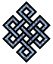#fail2ban bad ip database: ip 112.175.238.63

### | ip database | live view | stats | report | help | api key:

 ip: 112.175.238.63 hostname: 112.175.238.63 country:[KR] Korea, Republic of first reported: 17.11.2018 02:59.08 GMT+0200 last reported: 18.12.2018 02:33.46 GMT+0200 time period: 30d 23h 34m 38s total reports: 10 reported by: 3 host(s) filter(s): ssh (7) ssh (3) tor exit node no badips.com db Lookup## port scan of '112.175.238.63':

[-hide]
```# Nmap 6.40 scan initiated Sat Nov 17 03:00:04 2018 as: /usr/bin/nmap -sU -sS -O 112.175.238.63
Nmap scan report for 112.175.238.63
Host is up (0.27s latency).
Not shown: 1000 open|filtered ports, 997 filtered ports
PORT    STATE  SERVICE
22/tcp  open   ssh
80/tcp  open   http
443/tcp closed https
No exact OS matches for host (If you know what OS is running on it, see http://nmap.org/submit/ ).
TCP/IP fingerprint:
OS:SCAN(V=6.40%E=4%D=11/17%OT=22%CT=443%CU=%PV=N%G=Y%TM=5BEF765F%P=x86_64-p
OS:c-linux-gnu)SEQ(SP=103%GCD=1%ISR=10B%TI=Z%CI=I%TS=U)SEQ(SP=103%GCD=1%ISR
OS:=10B%TI=Z%TS=U)OPS(O1=M5B4NNSNW7%O2=M5B4NNSNW7%O3=M5B4NW7%O4=M5B4NNSNW7%
OS:O5=M5B4NNSNW7%O6=M5B4NNS)WIN(W1=7210%W2=7210%W3=7210%W4=7210%W5=7210%W6=
OS:7210)ECN(R=Y%DF=Y%TG=40%W=7210%O=M5B4NNSNW7%CC=Y%Q=)T1(R=Y%DF=Y%TG=40%S=
OS:O%A=S+%F=AS%RD=0%Q=)T2(R=N)T3(R=N)T4(R=Y%DF=Y%TG=40%W=0%S=A%A=Z%F=R%O=%R
OS:D=0%Q=)T5(R=Y%DF=Y%TG=40%W=0%S=Z%A=S+%F=AR%O=%RD=0%Q=)T6(R=Y%DF=Y%TG=40%
OS:W=0%S=A%A=Z%F=R%O=%RD=0%Q=)T7(R=N)U1(R=N)IE(R=N)

OS detection performed. Please report any incorrect results at http://nmap.org/submit/ .
# Nmap done at Sat Nov 17 03:01:03 2018 -- 1 IP address (1 host up) scanned in 61.46 seconds
```
```Σ = 44 | Δt = 0.0077559947967529s
```